The Mixed Integer Linear Programming Solver

Branch-and-Bound Algorithm

The branch-and-bound algorithm, first proposed by Land and Doig (1960), is an effective approach to solving mixed integer linear programs. The following discussion outlines the approach and explains how to enhance its progress by using several advanced techniques.

The branch-and-bound algorithm solves a mixed integer linear program by dividing the search space and generating a sequence of subproblems. The search space of a mixed integer linear program can be represented by a tree. Each node in the tree is identified with a subproblem derived from previous subproblems on the path that leads to the root of the tree. The subproblem (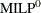) associated with the root is identical to the original problem, which is called (MILP), given in the section Overview: MILP Solver.

The linear programming relaxation (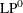) of () can be written as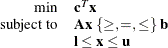The branch-and-bound algorithm generates subproblems along the nodes of the tree by using the following scheme. Consider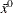, the optimal solution to (), which is usually obtained by using the dual simplex algorithm. If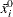is an integer for all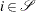, thenis an optimal solution to (MILP). Suppose that for some,is nonintegral. In that case the algorithm defines two new subproblems (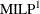) and (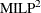), descendants of the parent subproblem (). The subproblem () is identical to () except for the additional constraint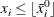and the subproblem () is identical to () except for the additional constraint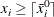The notation
represents the largest integer that is less than or equal to
, and the notation
represents the smallest integer that is greater than or equal to. The two preceding constraints can be handled by modifying the bounds of the variable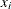rather than by explicitly adding the constraints to the constraint matrix. The two new subproblems do not haveas a feasible solution, but the integer solution to (MILP) must satisfy one of the preceding constraints. The two subproblems thus defined are called active nodes in the branch-and-bound tree, and the variableis called the branching variable.

In the next step the branch-and-bound algorithm chooses one of the active nodes and attempts to solve the linear programming relaxation of that subproblem. The relaxation might be infeasible, in which case the subproblem is dropped (fathomed). If the subproblem can be solved and the solution is integer feasible (that is,is an integer for all), then its objective value provides an upper bound for the objective value in the minimization problem (MILP); if the solution is not integer feasible, then it defines two new subproblems. Branching continues in this manner until there are no active nodes. At this point the best integer solution found is an optimal solution for (MILP). If no integer solution has been found, then (MILP) is integer infeasible. You can specify other criteria to stop the branch-and-bound algorithm before it processes all the active nodes; see the section Controlling the Branch-and-Bound Algorithm for details.

Upper bounds from integer feasible solutions can be used to fathom or cut off active nodes. Since the objective value of an optimal solution cannot be greater than an upper bound, active nodes with lower bounds higher than an existing upper bound can be safely deleted. In particular, ifis the objective value of the current best integer solution, then any active subproblems whose relaxed objective value is greater than or equal tocan be discarded.

It is important to realize that mixed integer linear programs are non-deterministic polynomial-time hard (NP-hard). Roughly speaking, this means that the effort required to solve a mixed integer linear program grows exponentially with the size of the problem. For example, a problem with 10 binary variables can generate in the worst case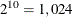nodes in the branch-and-bound tree. A problem with 20 binary variables can generate in the worst case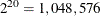nodes in the branch-and-bound tree. Although it is unlikely that the branch-and-bound algorithm has to generate every single possible node, the need to explore even a small fraction of the potential number of nodes for a large problem can be resource-intensive.

A number of techniques can speed up the search progress of the branch-and-bound algorithm. Heuristics are used to find feasible solutions, which can improve the upper bounds on solutions of mixed integer linear programs. Cutting planes can reduce the search space and thus improve the lower bounds on solutions of mixed integer linear programs. When using cutting planes, the branch-and-bound algorithm is also called the branch-and-cut algorithm. Preprocessing can reduce problem size and improve problem solvability. The MILP solver in PROC OPTMODEL employs various heuristics, cutting planes, preprocessing, and other techniques, which you can control through corresponding options.